# gscatter

## 语法

``gscatter(x,y,g)``
``gscatter(x,y,g,clr,sym,siz)``
``gscatter(x,y,g,clr,sym,siz,doleg)``
``gscatter(x,y,g,clr,sym,siz,doleg,xnam,ynam)``
``gscatter(ax,___)``
``h = gscatter(___)``

## 说明

``gscatter(x,y,g)` 创建 `x` 和 `y` 的散点图，按 `g` 分组。输入 `x` 和 `y` 是大小相同的向量。`

``gscatter(x,y,g,clr,sym,siz)` 为每个组指定标记颜色 `clr`、符号 `sym` 和大小 `siz`。`
``gscatter(x,y,g,clr,sym,siz,doleg)` 控制是否在图上显示图例。默认情况下 `gscatter` 会创建图例。`

``gscatter(x,y,g,clr,sym,siz,doleg,xnam,ynam)` 指定用于 x 轴和 y 轴标签的名称。如果不提供 `xnam` 和 `ynam`，并且 `x` 和 `y` 输入是带名称的变量，则 `gscatter` 用变量名称对坐标区加标签。`

``gscatter(ax,___)` 使用由坐标区对象 `ax` 指定的绘图坐标区。将 `ax` 指定为第一个输入参数，后跟先前语法中的任意输入参数组合。`

``h = gscatter(___)` 返回与 `g` 中的组对应的图形句柄。您可以为 `clr`、`sym` 和 `siz` 传入 `[]`，以使用其默认值。`

## 示例

`load carsmall`

`gscatter(Displacement,Horsepower,Model_Year)`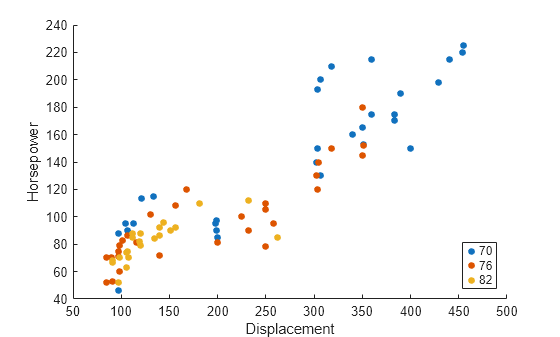`load discrim`

```gscatter(ratings(:,1),ratings(:,2),group,'br','xo') xlabel('climate') ylabel('housing')```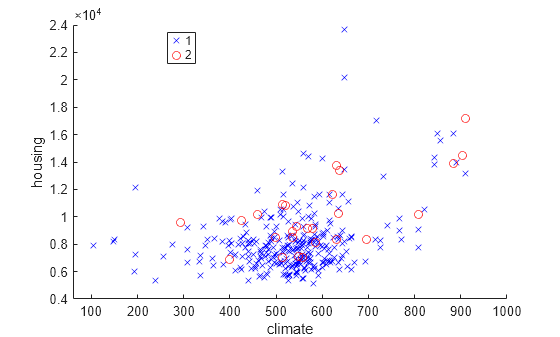`load hospital`

```x = hospital.Age; y = hospital.Weight; g = {hospital.Sex,hospital.Smoker}; gscatter(x,y,g,'rkgb','o*',8,'on','Age','Weight') legend('Location','northeastoutside')```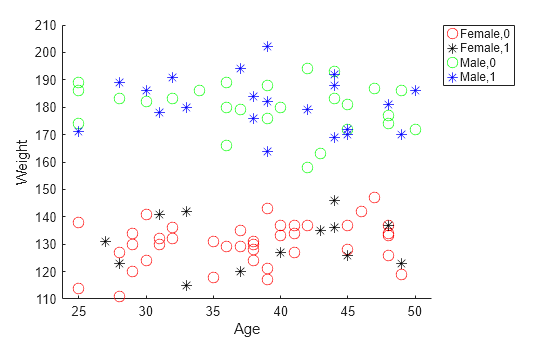```load carsmall color = lines(6); % Generate color values ax1 = subplot(1,2,1); % Left subplot gscatter(ax1,Acceleration,MPG,Model_Year,color(1:3,:)) title(ax1,'Left Subplot (Model Year)') ax2 = subplot(1,2,2); % Right subplot gscatter(ax2,Acceleration,MPG,Cylinders,color(4:6,:)) title(ax2,'Right Subplot (Cylinders)')```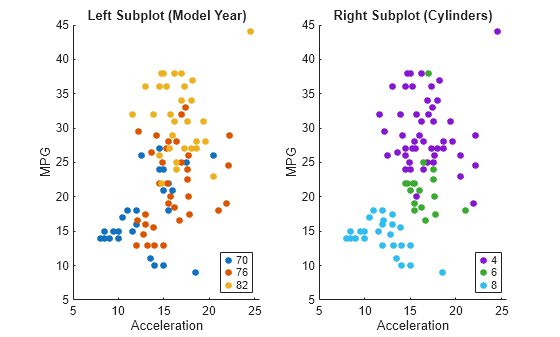```load('lidar_subset.mat') loc = lidar_subset;```

```xBound = 20; % in meters yBound = 20; % in meters zLowerBound = 0; % in meters```

```indices = loc(:,1) <= xBound & loc(:,1) >= -xBound ... & loc(:,2) <= yBound & loc(:,2) >= -yBound ... & loc(:,3) > zLowerBound; loc = loc(indices,:);```

```D = pdist2(loc,loc); idx = dbscan(D,2,50,'Distance','precomputed');```

```numGroups = length(unique(idx)); clr = hsv(numGroups); gscatter(loc(:,1),loc(:,2),idx,clr) xlabel('x') ylabel('y')```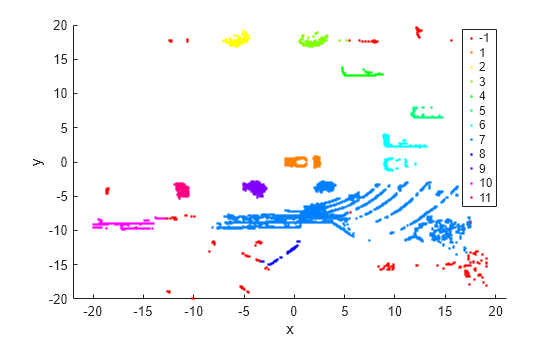`load carbig`

`h = gscatter(Acceleration,MPG,Origin)`
```h = 7x1 Line array: Line (USA) Line (France) Line (Japan) Line (Germany) Line (Sweden) Line (Italy) Line (England) ```

`jgroup = h(3)`
```jgroup = Line (Japan) with properties: Color: [0.9290 0.6940 0.1250] LineStyle: 'none' LineWidth: 0.5000 Marker: '.' MarkerSize: 15 MarkerFaceColor: 'none' XData: [15 14.5000 14.5000 14 19 18 15.5000 13.5000 17 ... ] YData: [24 27 27 25 31 35 24 19 28 23 27 20 22 18 20 31 32 ... ] Show all properties ```

`Japan` 组的标记颜色更改为黑色。

`jgroup.Color = 'k';`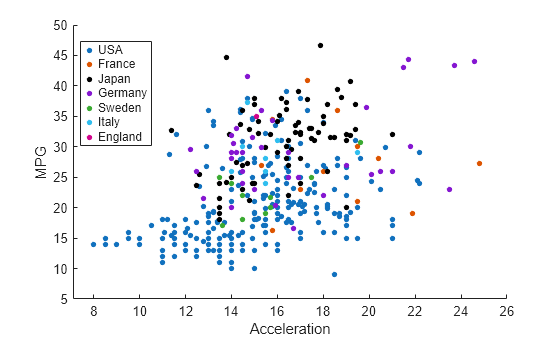## 输入参数

x 轴值，指定为数值向量。`x` 必须与 `y` 具有相同的大小。

y 轴值，指定为数值向量。`y` 必须与 `x` 具有相同的大小。

`g` 中的行数必须等于 `x` 的长度。

`'r'``[1 0 0]``'g'``[0 1 0]``'b'``[0 0 1]``'c'``[0 1 1]``'m'``[1 0 1]``'y'``[1 1 0]``'k'``[0 0 0]``'w'``[1 1 1]`RGB 三元组外观
`[0 0.4470 0.7410]``[0.8500 0.3250 0.0980]``[0.9290 0.6940 0.1250]``[0.4940 0.1840 0.5560]``[0.4660 0.6740 0.1880]``[0.3010 0.7450 0.9330]``[0.6350 0.0780 0.1840]``clr` 的默认值为包含 MATLAB 默认颜色的 RGB 三元组矩阵。

`'o'`圆形
`'+'`加号
`'*'`星号
`'.'`
`'x'`叉号
`'s'`正方形
`'d'`菱形
`'^'`上三角
`'v'`下三角
`'>'`右三角
`'<'`左三角
`'p'`五角星（五角形）
`'h'`六角星（六角形）
`'none'`无标记

x 轴标签，指定为字符向量或字符串标量。

y 轴标签，指定为字符向量或字符串标量。